# Python Program to Find Largest of Two Numbers

This article is created to cover some programs in Python, that find and prints largest or greatest between two numbers entered by user. Here are the list of approaches to do the job:

• Find Largest of Two Numbers using if-else
• Using if only
• Using user-defined Function

## Find Largest of Two Numbers using if-else

To find largest or greatest of two numbers in Python, you have to ask from user to enter any two numbers, then using if-else statement, find and print the largest one as shown in the program given below:

The question is, write a Python program to find largest between two numbers using if-else. Here is its answer:

```print("Enter Two Numbers: ")
numOne = int(input())
numTwo = int(input())

if numOne>numTwo:
print("\nLargest Number =", numOne)
else:
print("\nLargest Number =", numTwo)```

Here is its sample run: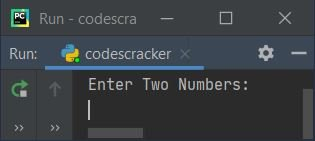Now supply inputs say 30 as first number, press `ENTER` key, then enter 40 as second number and press `ENTER` key to find and print largest of two numbers like shown in the snapshot given below: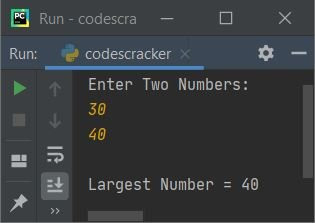The dry run of above program with same user input as provided in sample run, goes like:

• Initial values, numOne=30 (entered by user), numTwo=40 (also entered by user)
• Now the condition (of if) numOne>numTwo or 30>40 evaluates to be false, therefore program flow goes inside the body of its counterpart, that is else, and prints the value of numTwo as largest number

#### Modified Version of Previous Program

This is the modified version of previous program. This program handles with invalid and equal inputs both. That is, when user enters two numbers like c and \$, or 4 as both first and second number:

```print("Enter Two Numbers: ", end="")
try:
numOne = int(input())
try:
numTwo = int(input())
print()
if numOne>numTwo:
print(numOne, ">", numTwo)
elif numTwo>numOne:
print(numTwo, ">", numOne)
else:
print(numOne, "=", numTwo)
except ValueError:
print("\nInvalid Input!")
except ValueError:
print("\nInvalid Input!")```

Here is its sample run with user input, 50 and 50 as first and second number: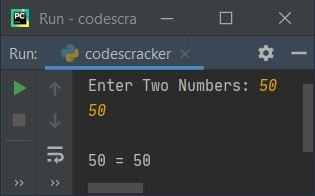And here is another sample run with two numbers input as 39 and 74: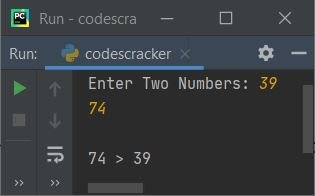## Find Largest of Two Numbers using if Only

This program is created using only if statement. Here using if, I've applied all three conditions. That is, whether first number is greater than second, whether second number is greater than first, or whether both numbers are equal.

```print("Enter Two Numbers: ", end="")
numOne = int(input())
numTwo = int(input())

if numOne>numTwo:
print("\nLargest Number =", numOne)
if numTwo>numOne:
print("\nLargest Number =", numTwo)
if numOne==numTwo:
print("\nBoth Numbers are Equal")```

Here is its sample run with user input 100 and 100 as both numbers: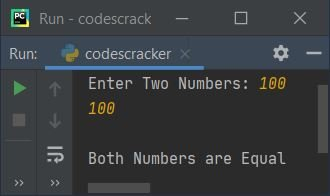## Find Largest of Two Numbers using Function

This is the last program of this article, created using a user-defined function named FindLargOfTwo(). It takes two arguments and returns 1, if first argument's value is greater, returns 2 if second argument's value is greater.

```def FindLargOfTwo(x, y):
if x>y:
return 1
elif x<y:
return 2

print("Enter Two Numbers: ", end="")
numOne = int(input())
numTwo = int(input())

res = FindLargOfTwo(numOne, numTwo)
if res==1:
print("\nLargest Number =", numOne)
elif res==2:
print("\nLargest Number =", numTwo)
else:
print("\nBoth Numbers are Equal")```

#### Same Program in Other Languages

Python Online Test

« Previous Program Next Program »